# 围观Razavi和Sansen打架——电流并联反馈

## 一、排兵布阵

### Sansen出招

Sansen《模集精粹》第14章反馈中介绍电流并联反馈时，要求输入电阻，和输出电阻，他的结果是：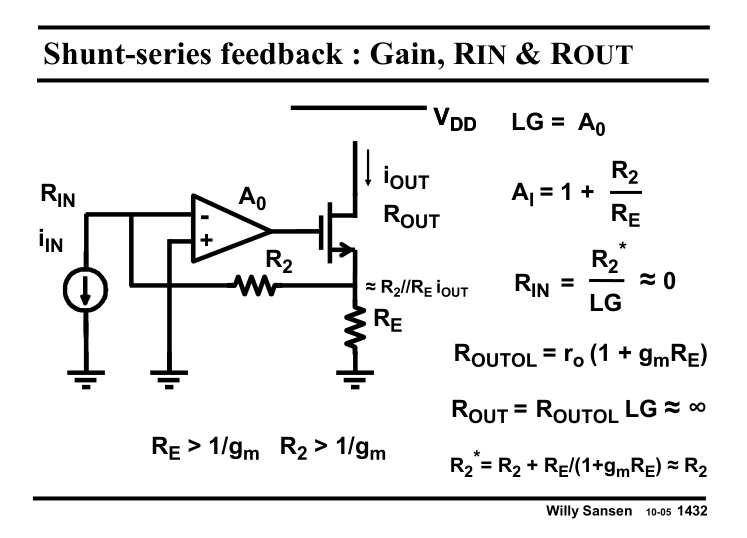$$R_{in}=\frac{R_2+R_E//\frac{1}{g_m}}{1+LG}\approx \frac{R_2}{A_0}（负载短路）$$
$$R_{out}=r_0(1+g_mR_E)(1+LG)\approx r_og_mR_EA_0（负载开路）$$

### Razavi出招

Razavi第八章的反馈也有这样的案例，同样是电流并联反馈。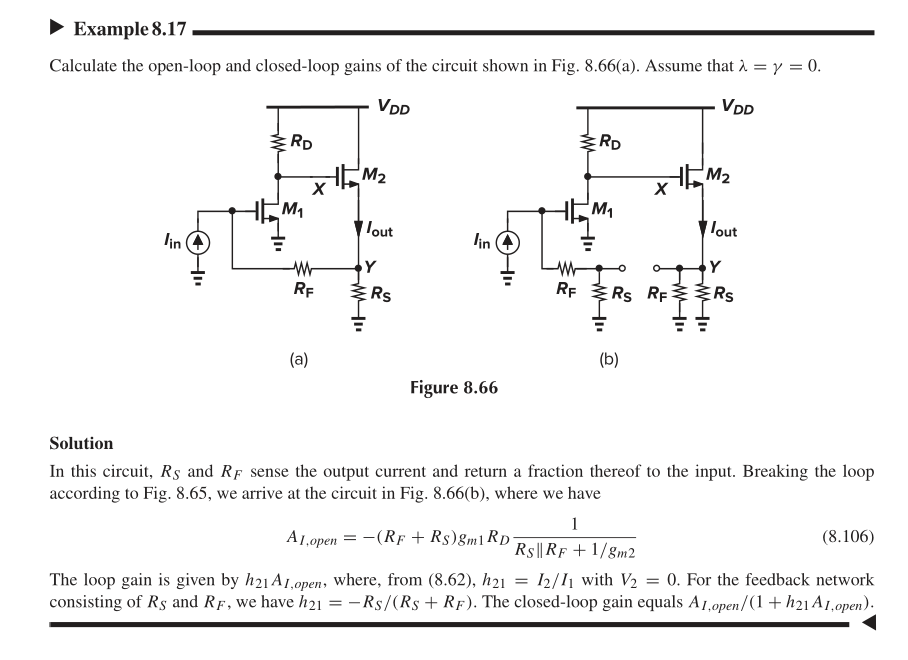$$R_{in}=\frac{R_2+R_E}{1+LG}\approx \frac{R_2+R_E}{A_0}（负载开路）$$
$$R_{out}=r_0(1+g_mR_E//R_2)(1+LG)\approx r_og_mR_E//R_2A_0（负载短路）$$

## 二、格雷劝架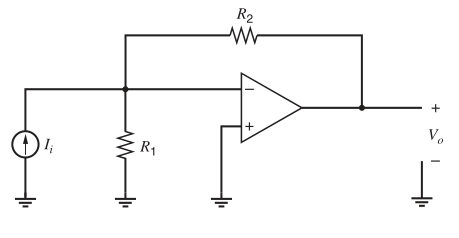Sansen用的是Return Ratio的方法，计算负载时需要用Blackman公式，而不是用二端口的开路负载乘上或除以环路增益。

Razavi用的是二端口的方法，该方法定义的环路增益与Return Ratio不同。

## 三、Razavi的二端口方法

### 1、打开环路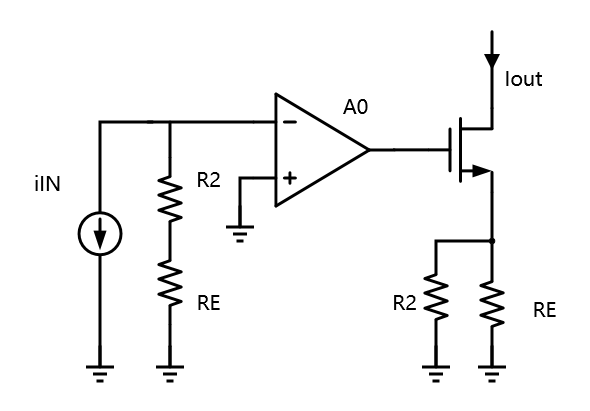### 2、分别计算开环增益$A_{open}$，反馈系数$F$

$$A_{open}=\frac{I_{out}}{I_{in}}=(R_2+R_E)A_0\frac{g_m}{1+g_mR_2//R_E}\approx\frac{(R_2+R_E)^2}{R_2R_E}A_0$$
$$F=\frac{I_F}{I_{out}}=\frac{I_F}{(1+R_2/R_E)I_F}=\frac{R_E}{R_E+R_2}$$

$$AF=\frac{R_2+R_E}{R_2}A_0$$

### 3、计算输入电阻、输出电阻

$$R_{in_{close}}=\frac{R_{in_{open}}}{1+AF}=\frac{R_2+R_E}{1+A_0\frac{R_2+R_E}{R_2}}\approx\frac{R_2}{A_0}$$
$$R_{out_{close}}=R_{out_{open}}(1+AF)=r_o(1+g_mR_2//R_E)(1+A_0\frac{R_2+R_E}{R_2})\approx r_og_mR_EA_0$$

## 四、Sansen的Return Ratio方法

### 1、断开环路，输入置零，求环路增益LG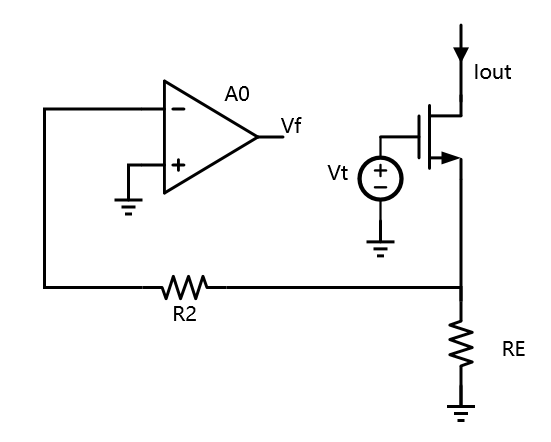$$LG=\frac{V_f}{V_t}=A_0$$

### 2、输入输出负载

Return Ratio的方法需要用Blackman公式计算输入输出负载：
$$Z_{port}=Z_{port}(k=0)[\frac{1+R(port\_shorted)}{1+R(port\_open)}]$$

#### 输出负载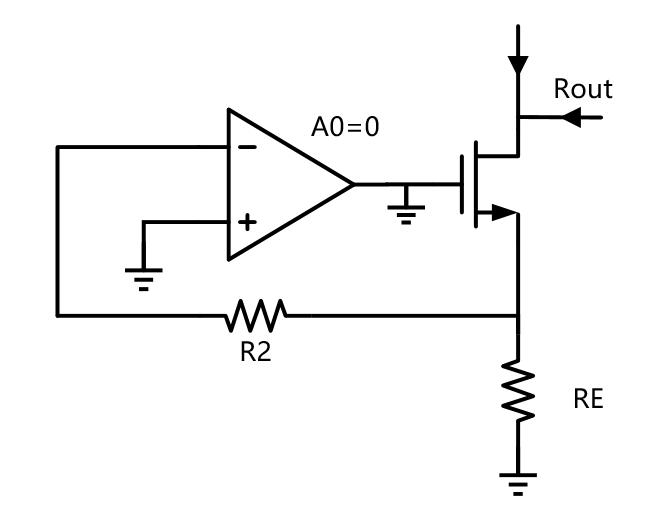$R(port\_shorted)$是将输出短路，恢复放大器增益时的环路增益；$R(port\_open)$是将输出开路，恢复放大器增益时的环路增益；两者的比值通常都是LG或者1/LG，具体要看反馈的类型。这里是输出是电流反馈，负载会提高，因此要乘以(1+LG)。
$$R_{out}=R_{outol}(1+LG)\approx r_og_mR_EA_0$$

#### 输入负载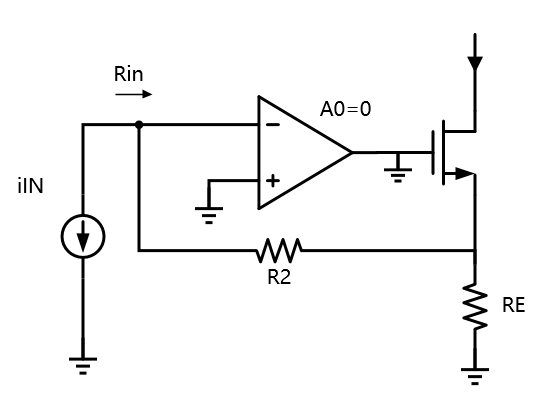$$R_{in}=\frac{R_{inol}}{1+LG}\approx \frac{R_2}{A_0}$$

## 五、总结

• 断开环路求$A_{open}$(需考虑负载)
• 计算环路增益$A_{open}F$
• 计算闭环增益，输入电阻，输出电阻

• 输入置零，断开环路，加测试信号求环路增益LG(特别注意这里的环路增益和二端口的环路增益定义不同)
• Blackman 公式计算输入输出电阻